## Filters

Sort by :
Clear All
Q

Q.2.    Find the area of any one door in your house.

suppose the length of door is 2m and breadth is 0.5 m  then the area of door =

Find the area of the floor of your classroom.

If floor length is 3m and breadth is 2m then

Find the perimeter of the following rectangles: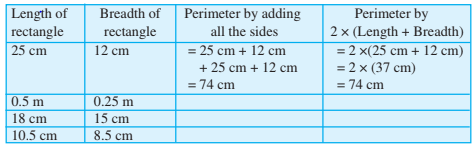Length of rectangle Breadth of rectangle Perimeter by adding all sides perimeter by  25 cm  12 cm =25+12+25+12=74 cm 0.5 cm 0.25cm =0.5+0.25+0.5+0.25=1.5cm 18 cm 15 cm =18+15+18+15=66 cm 10.5 cm 8.5 cm =10.5+8.5+10.5+8.5=38 cm

Find the perimeter of the following figures: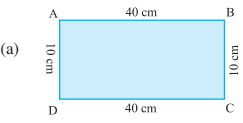Perimeter = AB + BC + CD + DA
= __+__+__+__
= ______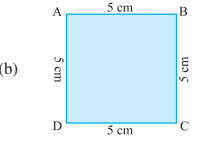Perimeter = AB + BC + CD + DA
= __ + __ + __+ __
= ______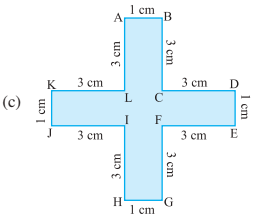Perimeter = AB + BC + CD + DE + EF + FG + GH +HI + IJ + JK + KL + LA
= __ + __ +__ + __ + __ +  __ + __ + __ +__+ __ + __ + __
= ______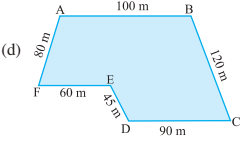Perimeter = AB + BC + CD + DE + EF+ FA
= __ + __ + __ + __ + __ + __
= ______

Find the perimeter of the following figures:                    Perimeter = AB + BC + CD + DA          = 40+10+40+10          = 100                Perimeter = AB + BC + CD + DA       =5+5+5+5=20                  Perimeter = AB + BC + CD + DE + EF + FG + GH +HI + IJ + JK + KL + LA                            =1+3+3+1+3+3+1+3+3+1+3+3=28 cm                       Perimeter = AB + BC + CD +...

Meera went to a park 150 m long and 80 m wide. She took one complete round on its boundary. What is the distance covered by her?

The park is 150m long and 80 m wide. The parks are of the shape of the rectangle. The distance covered by Meera = perimeter of park = 2 (150+80)= 460 m

Q 12.     How many tiles whose length and breadth are 12 cm and 5 cm respectively will be needed to fit in a rectangular region whose length and breadth are respectively:

(a) 100 cm and 144 cm
(b) 70 cm and 36 cm.

Given that, the length and breadth of the tiles are 12 cm and 5 cm Therefore, the area of the tiles  = length  breadth = 12 cm  5 cm = 60 cm2 Now, (a) For 100 cm and 144 cm rectangular region, Area of region =  length  breadth                         = 14,400 cm2 Therefore, the number of tiles needed =  tiles (b)  For 70 cm and 36 cm rectangular region, Area of region = ...

Q 11.     Split the following shapes into rectangles and find their areas. (The measures are given in centimetres)(a) Split the given figure into a small rectangle, as shown here; Now, the area of the rectangle (I) =  = 12  2 =   the area of the rectangle (II) =  = 8  2=   Therefore, Total area = (b) Split the given figure into a small rectangle, as shown here; Now, the area of the rectangle (I) =  = 7 7 = the area of the rectangle (II) =  = 21  7= Now, the area of the rectangle (III) =  = 7 7 =...

Q 10.     By splitting the following figures into rectangles, find their areas (The measures are given in centimetres).(a) Split the given figures into small rectangular parts as shown here; Now, the area of the rectangle (I) =  = 4  3 = 12  the area of the rectangle (II) =  = 3  2= 6  the area of the rectangle (III) =  = 4  1 = 4  the area of the rectangle (IV) =  = 3  2 = 6  So, the total area of the figure = sum area of all rectangles                                                  = (12 + 6 + 4 +...

Q 9.     Five square flower beds each of sides 1 m are dug on a piece of land 5 m long and 4 m wide. What is the area of the remaining part of the land?

Given that, Length and breadth of the land are 5m and 4 m. Side of the square flower bed = 1m Therefore the area of the bed = 1  So, Area of 5 square flower bed = 5  Area of the and = length  breadth                          =  Thus, the area of the remaining part of the land = Area of land - area of the flower bed                                                                          ...

Q 8.     A floor is 5 m long and 4 m wide. A square carpet of sides 3 m is laid on the floor. Find the area of the floor that is not carpeted.

Given that, Length of the floor = 5 m and its width = 4 m Therefore,  the area of the floor = length  breadth And, side of the square carpet = 3m Therefore,  the area of the square carpet =  = 3m  3m = 9m2 Thus, the area of the floor which is not covered with carpet =

Q 7.     A room is 4 m long and 3 m 50 cm wide. How many square metres of carpet is needed to cover the floor of the room?

Given that, Length of the room = 4 m And it's breadth = 3.5 m Area of the room = = length  breadth                                                              =  Hence the area of carpet needed is

Q 6.     A table-top measures 2 m by 1 m 50 cm. What is its area in square metres?

Given that, Length of the table-top = 2m and it's breadth = 1.5 m Therefore, the area of the tabletop  = length  breadth

Q 5.     What is the cost of tiling a rectangular plot of land 500 m long and 200 m wide at the rate of rupees 8 per hundred sq m.?

Given that, Length of the rectangular plot = 500m  and the width = 200 m fencing cost  = Rs 8 per hundred sq. meter Therefore, the area of the plot   =  Now, Total rate of tiling of 100,1000 m2   =                                                                   = Rs 8000

Q 4.     The area of a rectangular garden 50 m long is 300 sq m. Find the width of the garden.

Given that, Area of the rectangular garden = 300 sq. m and the length = 50 m Therefore, the Width of the garden =                                                           = Hence the width of the garden is 6 m

Q 3.     The length and breadth of three rectangles are as given below :

(a) 9 m and 6 m                 (b) 17 m and 3 m                 (c) 4 m and 14 m

Which one has the largest area and which one has the smallest?

We know that area of a rectangle = length  breadth Therefore, (a) Here length is 9m and the breadth of the rectangle is 6m        So, the area of the rectangle        =  (b) Here length is 17 m and the breadth of the rectangle is 3m        So, the area of the rectangle         =  (c) Here length is 4m and the breadth of the rectangle is 14m        So, the area of the rectangle       ...

Q 2.     Find the areas of the squares whose sides are :

(a) 10 cm                 (b) 14 cm             (c) 5 m

It is known that area of a square =  (a) The length of a side of a square = 10cm So, the area =  (b)The length of a side of a square = 14cm So, the area = (c) The length of a side of a square = 5m So, the area =

Q 1.       Find the areas of the rectangles whose sides are :

(a) 3 cm and 4 cm
(b) 12 m and 21 m
(c) 2 km and 3 km
(d) 2 m and 70 cm

We know that area of a rectangle = length  breadth Therefore, (a) Here length is 3cm and the breadth of the rectangle is 4cm        So, the area of the rectangle          =  (b) Here length is 12m and the breadth of the rectangle is 21m        So, the area of the rectangle          =  (c) Here length is 3km and the breadth of the rectangle is 3km        So, the area of the...

Q 1.     Find the areas of the following figures by counting square:Area of 1 square = 1sq. unit (a) Number of total squares = 9       Therefore, the area of 9 squares = 9 sq. units (b) In fig (b) the total number of square =  5       Therefore, are = 5 sq units (c) In fig (c) the number of squares = 4      So, the area is 4 sq. units (d) In fig (d) number of squares = 8       Therefore, area = 8 sq. units (e) Total number of square = 10       therefore,...

Q 17.      Avneet buys 9 square paving slabs, each with a side of . He lays them in the form of a square.

(a) What is the perimeter of his arrangement ?

(b) Shari does not like his arrangement. She gets him to lay them out like a cross. What is the perimeter of her arrangement?

(c) Which has greater perimeter?

(d) Avneet wonders if there is a way of getting an even greater perimeter. Can you find a way of doing this?Given that, Length of each slab =  So, the length of the square =  (a) The first figure is a square So, the perimeter of the square = 4  side                                                    =  (b) The perimeter of the 2nd figure = Sum of all the sides =  = 10 m (c) Figure (ii) has a greater perimeter than fig (i) (d) Arrange all the slab in horizontally, it forms a rectangle whose...

Q 16.     What is the perimeter of each of the following figures? What do you infer from the answers?(a) The perimeter of the square       = Sum of all the four side = 4   side       = 4   25       = 100 cm (b)The perimeter of the rectangle = SUm of all the sides = 2  [length + breadth ) = 2  (20 + 30) = 2  50 = 100 (c)The perimeter of the rectangle = Sum of all the sides = 2  [length + breadth ] = 2  (40 + 10) = 2  50 = 100 cm (d)The perimeter of the triangle = Sum of all three...
Exams
Articles
Questions